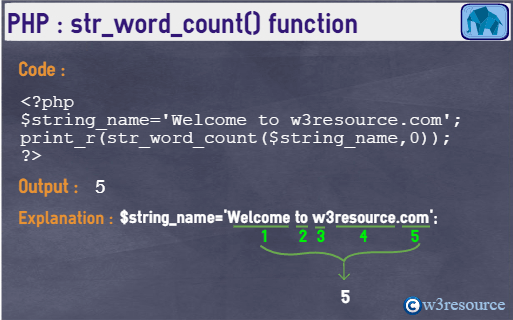# PHP: str_word_count() function

## Description

The str_word_count() function is used to count the number of words in a string.

Version:

(PHP 4 and above)

Syntax:

`str_word_count(string_name, nformat, add_chars)`

Parameter:

Name Description Required /
Optional
Type
string_name The input string. Required String
nformat Specifies the return value of the str_word_count() function.
Supported values :
0 - returns the number of words found.
1 - returns an array contains all the words found within the specified string.
2 - returns an associative array, where the key is the numeric position of the word inside the string and the value is the actual word itself.
Optional Integer
add_chars A list of additional characters which will be considered as 'word'. Optional String

Return value:

An array or an integer, depending on the format chosen.

Value Type: Mixed.

*Mixed: Mixed indicates that a parameter may accept multiple (but not necessarily all) types.

Pictorial PresentationExample:

``````<?php
\$string_name='Welcome to w3resource.com';
print_r(str_word_count(\$string_name,0));
echo '<br>';
print_r(str_word_count(\$string_name,1));
echo '<br>';
print_r(str_word_count(\$string_name,2));
?>
``````

Output :

```5
Array  (       => Welcome       => to       => w       => resource       => com  )
Array  (       => Welcome       => to       => w       => resource       => com  )```

View the example in the browser

PHP Function Reference

Previous: str_split
Next: strcasecmp

﻿

## PHP: Tips of the Day

Members of objects or classes can be accessed using the object operator (->) and the class operator (::).

Example:

```class MyClass {
public \$a = 1;
public static \$b = 2;
const C = 3;
public function d() { return 4; }
public static function e() { return 5; }
}
\$object = new MyClass();
var_dump(\$object->a); // int(1)
var_dump(\$object::\$b); // int(2)
var_dump(\$object::C); // int(3)
var_dump(MyClass::\$b); // int(2)
var_dump(MyClass::C); // int(3)
var_dump(\$object->d()); // int(4)
var_dump(\$object::d()); // int(4)
var_dump(MyClass::e()); // int(5)
\$classname = "MyClass";
var_dump(\$classname::e()); // also works! int(5)
```

Note that after the object operator, the \$ should not be written (\$object->a instead of \$object->\$a). For the class operator, this is not the case and the \$ is necessary. For a constant defined in the class, the \$ is never used.

Also note that var_dump(MyClass::d()); is only allowed if the function d() does not reference the object:

```class MyClass {
private \$a = 1;
public function d() {
return \$this->a;
}
}
\$object = new MyClass();
var_dump(MyClass::d()); // Error!
```

This causes a 'PHP Fatal error: Uncaught Error: Using \$this when not in object context'

These operators have left associativity, which can be used for 'chaining':

```class MyClass {
private \$a = 1;

\$this->a += \$a;
return \$this;
}

public function get() {
return \$this->a;
}
}
\$object = new MyClass();
```

These operators have the highest precedence (they are not even mentioned in the manual), even higher that clone. Thus:

```class MyClass {
private \$a = 0;
\$this->a += \$a;
return \$this;
}
public function get() {
return \$this->a;
}
}
\$o1 = new MyClass();
var_dump(\$o1->get()); // int(2)
var_dump(\$o2->get()); // int(2)
```

The value of \$o1 is added to before the object is cloned!

Note that using parentheses to influence precedence did not work in PHP version 5 and older (it does in PHP 7):

```// using the class MyClass from the previous code
\$o1 = new MyClass();
\$o2 = (clone \$o1)->add(2); // Error in PHP 5 and before, fine in PHP 7
var_dump(\$o1->get()); // int(0) in PHP 7
var_dump(\$o2->get()); // int(2) in PHP 7
```